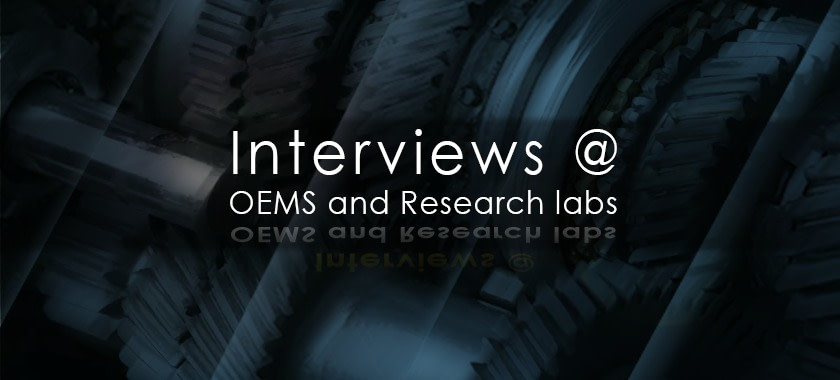# Interviews at OEMs and Research labs – Connecting Theory to PracticeAn OEM or a research interview is designed to test the ability of the candidate to solve a problem by applying physics-driven thinking skills. Unless the interviews are for people who would develop mechanical methods/mathematical models for high-end research interviews mostly check fundamentals, application and thinking skills.

### Entrant Level- Typical OEM

Expectation: Understanding of basic loads and ability to calculate stress in simple components

### Interviewer

If the bolt hole size is greater than the bolt size then compute the preload needed to sustain the weight of the bracket. Neglect the moment due to the weight of the bracket at the joint

### Candidate

Analysis: It is easy to visualise that when bolt has clearance it does not take any load. It is the friction available between wall and the bracket and the pre-load that go to constitute the balancing force for weight

If there are  N bolts and if the preload is P then we have### Engineers' note:

We assume preload applies uniform pressure. Secondly we also need to note that preload is measured by measuring the stretch of the bolt traditionally and 90% of the pre-load torque is consumed by friction.

### Entrant Level- Typical Research

Expectation: Understanding of basic loads and ability to observe unobvious facets

### Interviewer

How do you account for shear stress variation across a circular cross section?

### Step 1

One must note that variation is parabolic hence we if we assume average shear stress a correction factor must be applied.

The shear correction factor is used to account for the parabolic variation of shear stress the cross-section of the beam or shaft. When we take average shear stress, it is load by actual cross-section area, where we assume constant shear stress across the depth. This does not account for shear stress variation and hence the area is corrected by these factors.

The General formula for shear stress variation is given by

### Step 2 - Quantification

Let us find the Average shear strain energy.

### Engineers' note:

The shear stress effect is significant only to thick beams, plates, and shells. However, for composites, the shear modulus in the transverse direction is much lower than that of the Young’s modulus along the longitudinal direction and hence effect of shear stress is very important.

### Fairly experienced

Expectation: Critical thinking skills for product design

### Interviewer

Analyze the buckling possibility of a blade. Shaft blade system is considered as shown below. Find if the blade buckles due to CF growth and thermal growth, considering the initial clearance. Account for elasticity of shaft. Consider the shaft to be massless.

### Step 1

The load developed by the constraint of the growth, beyond the cold clearance, contributes to the compressive buckling load. Hence, net growth beyond cold clearance must be computed. Elasticity of the shaft makes the situation worse.

Observation: For assessing the growth of the blade, the centrifugal load is considered without the shaft’s elastic deflection.

Hence, the blade is likely to buckle if centrifugal growth +Thermal growth +elastic deflection of the shaft > cold clearance.

### Step 3 - Analysis

• What is assumed here is the growth of the outer casing is not significant.
• One could assume fixed and pinned condition to compute the buckling load and hence the difference in deflections. The Euler’s buckling load formula could be used.
• Worst product temperature must be used for growth calculations

### Did you know?

• Does the single crystal blade suffer higher growth in the radial direction owing to smaller Young’s modulus?
• Does the typical turbine disc suffer more centrifugal growth than the blades?
• Have you used thermal and centrifugal growths in tolerance-stackup analysis?

0
0